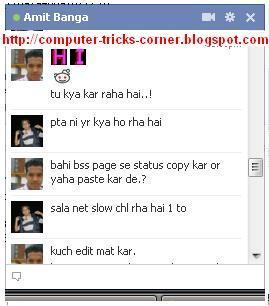# Send Colorful Text In Facebook ChatFacebook is  #1 social networking site where you can find almost all of your family members,   Friends, Classmates, etc. Some of facebook users only sign up for chatting and video calling with distant people. This is a trick to make your text colorful in facebook chat to impress the person you are chatting with. It works on all browsers and so easy to do.Note: This trick only works on Computer or Laptop no where else this works. In mobile it will show code in place of letter. So if you and the person you are chatting with must be from Computer or Laptop..

How many times you start chat in facebook by " Hi " ..? ?? I think most of time..! now in this post i'll tell you how you can add colorfull text in facebook chat without any software and addon just to impress your friends .! !!

First of all let me show you a example :-
In this chat box you can see HI in pink color it looks nice, at-least batter then that ordinary boring " hi " .So want to know how to do it:-

How to write HI in pink color :-

just copy the below code :-

[] []
and paste it in chat window and simply hit enter..!

### How To Send Colorful Text In Facebook Chat

2. Select the online person you want to send colorful text.
3. Copy the code as given below according to your requirement.
4. Paste it on Chat box.
5. Hit enter and see magic!

Like in above case we wrote HI

HI :-   []  []
H                     I

Note: If you want to type HI in different color keep space between two Codes.
[] [] - Right Way.
[][]  - Wrong Way.

### Codes To Make Colorful Text

[] = C
[] = D
[] = E
[] = F
[] = G
[] = H
[] = I
[] = J
[] = K
[] = L
[] = M
[] = N
[] = O
[] = P
[] = Q
[] = R
[] = S
[] = T
[] = U
[] = V
[] []  = W
[] = X
[] = Y
[] = Z

[] = Bingung
[] = Thumbs
[] = Nope
[] = Capede
[] = Alay
[] = Trollface
[[foreveralonecomics]] = Forever alone.
[[MegustaMeMe]] = Me Gusta.
[] = Yao.
[] = LoL.
[[FapFapFapFap.B]] = Fap Fap Fap Fap.
[] = Y U NO.
[] = Nothing to do here.
[] = Poker Face.
[[yahoo]] = Yahoo
[[amazon]] = Amazon
[[opera]] = Opera
[[internetexplorer]] = Internet Explorer
[[flock]] =  Flock
[[microsoft]] =  Microsoft
[[microsoftword]] = Microsoft Word
[[microsoftexcel]] = Microsoft Excel
[[microsoftpowerpoint]] = Microsoft PowerPoint
[[megaxus]] = Megaxus
[[grandchase]] = Grandchase
[[indovision]] = Indovision
[[history]] = History
[[sony]] = Sony
[[nokia]] = Nokia
[[telkomsel]] = Telkomsel
[[windows]] = Windows
[[transformer]] = Transformer
[[itunes]] = iTunes
[[photoshop]] = Photoshop

How it works:-
Till then assume that these are codes . :D :-)
[]  = A
[]  = B
[]  = C
[]  = D
[]  = E
[]  = F
[]  = G
[]  = H
[]  = I
[]  = J
[]  = K
[]  = L
[]  = M
[]  = N
[]  = O
[]  = P
[]  = Q
[]  = R
[]  = S
[]  = T
[]  = U
[]  = V
[]  = W
[]  = X
[]  = Y
[]        = Z

So you can create any word using these codes like:-

COMPUTER :- [] [] [] [] [] [] []  []

TRICKS :-  [] [] [] [] [] []

CORNER :- [] []  [] []  [] []

Other useful codes:-

BIE :- [] [] []

NOTE:-
• Above codes will work only on Facebook chat and private messages . Codes will not work on status updates.!
• While creating a word using above codes remember to give space between 2 codes for eg:-
• HI :-   [][] ( WRONG METHOD  there is no space between 2 codes and it will appear as a code)
• HI :-   []  [] ( RIGHT METHOD )
• This will not work while using facebook on mobile